# PAMR: Passive aggressive mean reversion strategy for portfolio selection

## Abstract

This article proposes a novel online portfolio selection strategy named “Passive Aggressive Mean Reversion” (PAMR). Unlike traditional trend following approaches, the proposed approach relies upon the mean reversion relation of financial markets. Equipped with online passive aggressive learning technique from machine learning, the proposed portfolio selection strategy can effectively exploit the mean reversion property of markets. By analyzing PAMR’s update scheme, we find that it nicely trades off between portfolio return and volatility risk and reflects the mean reversion trading principle. We also present several variants of PAMR algorithm, including a mixture algorithm which mixes PAMR and other strategies. We conduct extensive numerical experiments to evaluate the empirical performance of the proposed algorithms on various real datasets. The encouraging results show that in most cases the proposed PAMR strategy outperforms all benchmarks and almost all state-of-the-art portfolio selection strategies under various performance metrics. In addition to its superior performance, the proposed PAMR runs extremely fast and thus is very suitable for real-life online trading applications. The experimental testbed including source codes and data sets is available at http://www.cais.ntu.edu.sg/~chhoi/PAMR/.

## Introduction

Portfolio Selection (PS) is a practical financial engineering problem that requires determining a strategy of investing wealth among a set of assets in order to achieve certain objectives, such as maximizing cumulative wealth or risk-adjusted return, in the long run. In this article, we investigate sequential portfolio selection (also termed online portfolio selection) strategies, which sequentially determine portfolios based on publicly available information.

Traditionally in finance, portfolios are often selected according to mean-variance theory (Markowitz 1952, 1959) or its variants, to trade off between return and risk. In recent years, this problem has also been actively studied from a learning to select portfolio perspective, with roots in the fields of machine learning, data mining, information theory and statistics. Rather than trading with a single stock using computational intelligence techniques, learning to select portfolio approach focuses on a portfolio, which consists of multiple assets/stocks. Several approaches for online portfolio selection, often characterized by machine learning formulations and effective optimization solutions, have been proposed in literature (Kelly 1956; Breiman 1961; Cover 1991; Ordentlich and Cover 1996; Helmbold et al. 1996; Borodin and El-Yaniv 1998; Borodin et al. 2000, 2004; Stoltz and Lugosi 2005; Hazan 2006; Györfi et al. 2006; Blum and Mansour 2007; Levina and Shafer 2008; Györfi et al. 2008). Despite being studied extensively, most approaches are limited in some aspects or the other.

Our goal of this work is to investigate a new online portfolio selection strategy that employs online learning techniques to exploit the financial markets. Some existing strategies adopt the trend following approach, that is, they assume that price relative will follow its historical trading days. However, this philosophy fails when price relatives do not go in any particular direction, but rather actively move within a range. So in this study, we exploit another well-known principle in finance, viz., mean reversion (Jegadeesh 1990), through an online machine learning framework. To this end, we propose a novel portfolio selection strategy named “Passive Aggressive Mean Reversion” (PAMR), which exploits the mean reversion property of financial markets by online passive aggressive learning (Crammer et al. 2006). PAMR’s key idea is to formulate a new loss function that can effectively exploit the mean reversion property, and then adopt passive aggressive online learning to search for optimal portfolio among the asset pool to maximize the cumulative return.

Under different scenarios, the proposed PAMR strategy either passively keeps last portfolio or aggressively approaches a new portfolio by following the mean reversion principle. By solving three well formulated optimization problems, we arrive at three simple portfolio update rules. It is interesting to find that the final portfolio update scheme reaches certain trade-offs between portfolio return and volatility risk, and explicitly reflects the mean reversion trading rule. Moreover, we propose a mixture algorithm, which mixes PAMR and other strategies, and show that the mixture can be universal if one universal strategy is included. The key advantages of PAMR are its highly competitive performance and fairly attractive computation time efficiency. Our extensive numerical experiments on various real datasets show that in most cases the proposed PAMR strategy is quite performance efficient in comparison to a number of state-of-the-art portfolio selection strategies under a variety of performance metrics. At the same time, the proposed strategy costs linear time with respect to the product of the number of stocks and trading days, and its computational time in back tests is orders of magnitude less than its competitors, showing its applicability to real-world large scale online applications.

1. 1.

We propose a new algorithm for online portfolio selection, named “Passive Aggressive Mean Reversion” (PAMR). To the best of our knowledge, it is the first portfolio selection strategy that exploits both the mean reversion property in finance and the powerful online passive aggressive learning technique in machine learning.

2. 2.

We propose a mixture algorithm to mix the proposed PAMR algorithms and other universal strategies, resulting in a theoretically guaranteed universal mixture strategy.

3. 3.

We analyze the final portfolio update scheme of PAMR and show that it is essentially related to certain trade-offs between portfolio return and volatility risk.

4. 4.

We conduct an extensive set of numerical experiments on a number of up-to-date datasets from various markets. The results show that in most cases the proposed PAMR strategy not only outperforms the benchmarks (including market index, best stock and challenging best constant rebalanced portfolio (Cover 1991) in hindsight), but also outperforms various state-of-the-art strategies under various performance metrics tested.

5. 5.

We also extend the proposed strategy to handle some practical issues for a real-life portfolio selection task, viz., transaction cost and margin buying, and show its practical viability through the extensive empirical study.

6. 6.

We show that the time complexity of the proposed algorithm is linear with respect to the number of stocks per trading day, and its empirical computational time in the back tests is quite competitive compared with the state of the arts, indicating the proposed strategy is suitable for online large-scale real applications.

The rest of the article is organized as follows. Section 2 formally states online portfolio selection problem. Section 3 reviews existing state-of-the-art approaches tackling this problem, and highlights their limitations. Section 4 presents our proposed PAMR strategy and analyzes the algorithm. Section 5 validates the effectiveness of PAMR by extensive empirical studies on historical financial markets. Finally, Sect. 6 summarizes this article and indicates future directions.

## Problem setting

Let us consider a financial market with m assets, over which we wish to invest. The changes of asset prices for n trading periods are represented by a sequence of non-negative, non-zero price relative vectors $${\mathbf{x}}_{1}, \ldots, {\mathbf{x}}_{n}\in{\mathbb{R}}_{+}^{m}$$. Let us use x n to denote such a sequence of vectors. The ith component of the tth vector x ti denotes the ratio of closing price to last closing price of the ith asset on the tth trading day, thus an investment in asset i on the tth trading day increases by a factor of x ti .

An investment in the market is specified by a portfolio vector b t =(b t1,…,b tm ), where b ti represents the proportion of wealth invested in the ith asset. Typically, we assume portfolio is self-financed and no margin/short is allowed, therefore each entry of a portfolio is non-negative and adds up to one, that is, b t ∈Δ m , where $${\Delta}_{m}= \{{\mathbf{b}} : {\mathbf {b}}\in {\mathbb{R}}_{+}^{m}, \sum_{i=1}^{m} b_{i} = 1 \}$$. The investment procedure is represented by a portfolio strategy, that is, a sequence of mappings $$\mathbf{b}_{1}= (\frac{1}{m}, \dots, \frac{1}{m} ), {\mathbf {b}}_{t}: {\mathbb{R}}_{+}^{m(t-1)}\rightarrow\Delta_{m}, t=2, 3, \ldots$$, where b t =b t (x 1,…,x t−1) is the portfolio used on the tth trading period given past market price relatives x t−1={x 1,…,x t−1}. Let us denote by b n the portfolio strategy for n trading periods.

For the tth trading day, an investment according to portfolio b t results in a portfolio daily return s t , that is, the wealth increases by a factor of $${\mathbf{s}}_{t}={\mathbf{b}}_{t}^{\top}{\mathbf{x}}_{t}=\sum_{i=1}^{m}b_{ti}x_{ti}$$. Since we use price relative, the investment results in multiplicative cumulative return. Thus, after n trading days, the investment according to a portfolio strategy b n results in portfolio cumulative wealth S n , which is increased by a factor of $$\prod_{t=1}^{n}{\mathbf{b}}_{t}^{\top} {\mathbf{x}}_{t}$$, that is,

$${\mathbf{S}}_{n} \bigl({\mathbf{b}}^{n}, {\mathbf{x}}^{n} \bigr) = {\mathbf{S}}_{0} \prod _{t=1}^n {\mathbf{b}}_{t}^{\top} {\mathbf{x}}_{t},$$

## References

• Abernethy, J., Agarwal, A., Barlett, P. L., & Rakhlin, A. (2009). A stochastic view of optimal regret through minimax duality. In Proceedings of annual conference on learning theory.

• Agarwal, A., & Hazan, E. (2005). New algorithms for repeated play and universal portfolio management. Technical report, Princeton University.

• Agarwal, A., Hazan, E., Kale, S., & Schapire, R. E. (2006). Algorithms for portfolio management based on the newton method. In Proceedings of the international conference on machine learning (pp. 9–16).

• Akcoglu, K., Drineas, P., & Kao, M.-Y. (2002). Fast universalization of investment strategies with provably good relative returns. In Automata, languages and programming (Vol. 2380, p. 782).

• Aldridge, I. (2009). High-frequency trading: a practical guide to algorithmic strategies and trading systems. Hoboken: Wiley.

• Belentepe, C. Y. (2005). A statistical view of universal portfolios. PhD thesis, University of Pennsylvania.

• Blum, A., & Kalai, A. (1999). Universal portfolios with and without transaction costs. Machine Learning, 35(3), 193–205.

• Blum, A., & Mansour, Y. (2007). From external to internal regret. Journal of Machine Learning Research, 8, 1307–1324.

• Borodin, A., & El-Yaniv, R. (1998). Online computation and competitive analysis. Cambridge: Cambridge University Press.

• Borodin, A., El-Yaniv, R., & Gogan, V. (2000). On the competitive theory and practice of portfolio selection (extended abstract). In Proceedings of the Latin American symposium on theoretical informatics (pp. 173–196).

• Borodin, A., El-Yaniv, R., & Gogan, V. (2004). Can we learn to beat the best stock. The Journal of Artificial Intelligence Research, 21, 579–594.

• Boyd, S., & Vandenberghe, L. (2004). Convex optimization. New York: Cambridge University Press.

• Breiman, L. (1961). Optimal gambling systems for favorable games. In Proceedings of the Berkeley symposium on mathematical statistics and probability (Vol. 1, pp. 65–78).

• Cao, L. J., & Tay, F. E. H. (2003). Support vector machine with adaptive parameters in financial time series forecasting. IEEE Transactions on Neural Networks, 14(6), 1506–1518.

• Cesa-Bianchi, N., & Lugosi, G. (2006). Prediction, learning, and games. New York: Cambridge University Press.

• Cesa-Bianchi, N., Conconi, A., & Gentile, C. (2004). On the generalization ability of on-line learning algorithms. IEEE Transactions on Information Theory, 50(9), 2050–2057.

• Cover, T. M. (1991). Universal portfolios. Mathematical Finance, 1(1), 1–29.

• Cover, T. M., & Gluss, D. H. (1986). Empirical bayes stock market portfolios. Advances in Applied Mathematics, 7(2), 170–181.

• Cover, T. M., & Ordentlich, E. (1996). Universal portfolios with side information. IEEE Transactions on Information Theory, 42(2), 348–363.

• Cover, T. M., & Thomas, J. A. (1991). Elements of information theory. New York: Wiley-Interscience.

• Crammer, K., & Singer, Y. (2003). Ultraconservative online algorithms for multiclass problems. Journal of Machine Learning Research, 3, 951–991.

• Crammer, K., Dekel, O., Keshet, J., Shalev-Shwartz, S., & Singer, Y. (2006). Online passive-aggressive algorithms. Journal of Machine Learning Research, 7, 551–585.

• Cross, J. E., & Barron, A. R. (2003). Efficient universal portfolios for past-dependent target classes. Mathematical Finance, 13(2), 245–276.

• Das, P., & Banerjee, A. (2011). Meta optimization and its application to portfolio selection. In Proceedings of international conference on knowledge discovery and data mining.

• Duchi, J., Shalev-Shwartz, S., Singer, Y., & Chandra, T. (2008). Efficient projections onto the l 1-ball for learning in high dimensions. In Proceedings of the international conference on machine learning (pp. 272–279).

• Exley, J., Mehta, S., & Smith, A. (2004). Mean reversion. Technical report, Faculty & Institute of Actuaries, Finance and Investment Conference, Brussels.

• Fink, M., Shalev-Shwartz, S., Singer, Y., & Ullman, S. (2006). Online multiclass learning by interclass hypothesis sharing. In Proceedings of the international conference on machine learning (pp. 313–320).

• Freund, Y., & Schapire, R. E. (1999). Large margin classification using the perceptron algorithm. Machine Learning, 37(3), 277–296.

• Gentile, C. (2001). A new approximate maximal margin classification algorithm. Journal of Machine Learning Research, 2, 213–242.

• Grinold, R., & Kahn, R. (1999). Active portfolio management: a quantitative approach for producing superior returns and controlling risk. New York: McGraw-Hill.

• Györfi, L., & Schäfer, D. (2003). Nonparametric prediction. In J. A. K. Suykens, G. Horváth, S. Basu, C. Micchelli, & J. Vandevalle (Eds.), Advances in learning theory: methods, models and applications (pp. 339–354). Amsterdam: IOS Press.

• Györfi, L., & Vajda, I. (2008). Growth optimal investment with transaction costs. In Proceedings of the international conference on algorithmic learning theory (pp. 108–122).

• Györfi, L., Lugosi, G., & Udina, F. (2006). Nonparametric kernel-based sequential investment strategies. Mathematical Finance, 16(2), 337–357.

• Györfi, L., Urbán, A., & Vajda, I. (2007). Kernel-based semi-log-optimal empirical portfolio selection strategies. International Journal of Theoretical and Applied Finance, 10(3), 505–516.

• Györfi, L., Udina, F., & Walk, H. (2008). Nonparametric nearest neighbor based empirical portfolio selection strategies. Statistics & Decisions, 26(2), 145–157.

• Hazan, E. (2006). Efficient algorithms for online convex optimization and their applications. PhD thesis, Princeton University.

• Hazan, E., & Seshadhri, C. (2009). Efficient learning algorithms for changing environments. In Proceedings of the international conference on machine learning (pp. 393–400).

• Hazan, E., Agarwal, A., & Kale, S. (2007). Logarithmic regret algorithms for online convex optimization. Machine Learning, 69(2–3), 169–192.

• Helmbold, D. P., Schapire, R. E., Singer, Y., & Warmuth, M. K. (1996). On-line portfolio selection using multiplicative updates. In Proceedings of the international conference on machine learning (pp. 243–251).

• Helmbold, D. P., Schapire, R. E., Singer, Y., & Warmuth, M. K. (1997). A comparison of new and old algorithms for a mixture estimation problem. Machine Learning, 27(1), 97–119.

• Hillebrand, E. (2003). Mean reversion models of financial markets. PhD thesis, University of Bremen.

• Hull, J. C. (2008). Options, futures, and other derivatives. Upper Saddle River: Prentice Hall.

• Jegadeesh, N. (1990). Evidence of predictable behavior of security returns. The Journal of Finance, 45(3), 881–898.

• Kalai, A., & Vempala, S. (2002). Efficient algorithms for universal portfolios. Journal of Machine Learning Research, 3, 423–440.

• Kelly, J. (1956). A new interpretation of information rate. Bell Systems Technical Journal, 35, 917–926.

• Kimoto, T., Asakawa, K., Yoda, M., & Takeoka, M. (1993). Stock market prediction system with modular neural networks. In Neural networks in finance and investing (p. 343–357).

• Kivinen, J., Smola, A. J., & Williamson, R. C. (2001). Online learning with kernels. In Proceedings of the annual conference on neural information processing systems.

• Latané, H. A. (1959). Criteria for choice among risky ventures. Journal of Political Economy, 67(2), 144–155.

• Levina, T., & Shafer, G. (2008). Portfolio selection and online learning. International Journal of Uncertainty, Fuzziness and Knowledge-Based Systems, 16(4), 437–473.

• Li, Y., & Long, P. M. (1999). The relaxed online maximum margin algorithm. In Proceedings of the annual conference on neural information processing systems.

• Li, B., Hoi, S. C. H., & Gopalkrishnan, V. (2011a). Corn: correlation-driven nonparametric learning approach for portfolio selection. ACM Transactions on Intelligent Systems and Technology, 2(3), 1–29. doi:10.1145/1961189.1961193

• Li, B., Hoi, S. C. H., Zhao, P., & Gopalkrishnan, V. (2011b). Confidence weighted mean reversion strategy for on-line portfolio selection. In Proceedings of the international conference on artificial intelligence and statistics (pp. 434–442).

• Lo, A. W., & MacKinlay, A. C. (1990). When are contrarian profits due to stock market overreaction? The Review of Financial Studies, 3(2), 175–205.

• Lu, C.-J., Lee, T.-S., & Chiu, C.-C. (2009). Financial time series forecasting using independent component analysis and support vector regression. Decision Support Systems, 47, 115–125.

• Magdon-Ismail, M., & Atiya, A. (2004). Maximum drawdown. Risk Magazine, 10, 99–102.

• Markowitz, H. (1952). Portfolio selection. The Journal of Finance, 7(1), 77–91.

• Markowitz, H. (1959). Portfolio selection: efficient diversification of investments. New York: Wiley.

• Michelot, C. (1986). A finite algorithm for finding the projection of a point onto the canonical simplex of ℝn. Journal of Optimization Theory and Applications, 50, 195–200.

• Moody, J., Wu, L., Liao, Y., & Saffell, M. (1998). Performance functions and reinforcement learning for trading systems and portfolios. Journal of Forecasting, 17, 441–471.

• Ordentlich, E., & Cover, T. M. (1996). On-line portfolio selection. In Proceedings of the annual conference on learning theory.

• Ottucsák, G., & Vajda, I. (2007). An asymptotic analysis of the mean-variance portfolio selection. Statistics & Decisions, 25, 63–88.

• Poterba, J. M., & Summers, L. H. (1988). Mean reversion in stock prices: evidence and implications. Journal of Financial Economics, 22(1), 27–59.

• Rosenblatt, F. (1958). The perceptron: a probabilistic model for information storage and organization in the brain. Psychological Review, 65, 386–407.

• Sharpe, W. F. (1963). A simplified model for portfolio analysis. Management Science, 9, 277–293.

• Sharpe, W. F. (1994). The sharpe ratio. Journal of Portfolio Management, 21(1), 49–58.

• Singer, Y. (1997). Switching portfolios. International Journal of Neural Systems, 8(4), 488–495.

• Stoltz, G., & Lugosi, G. (2005). Internal regret in on-line portfolio selection. Machine Learning, 59(1–2), 125–159.

• Tay, F. E. H., & Cao, L. (2001). Application of support vector machines in financial time series forecasting. Omega, 29(4), 309–317.

• Tsang, E., Yung, P., & Li, J. (2004). Eddie-automation, a decision support tool for financial forecasting. Decision Support Systems, 37, 559–565.

• Zhao, P., Hoi, S. C. H., & Jin, R. (2011). Double updating online learning. Journal of Machine Learning Research, 12, 1587–1615.

## Acknowledgements

This paper was fully supported by Singapore MOE Tier-1 Research Grant (RG67/07).

## Author information

Authors

### Corresponding author

Correspondence to Steven C. H. Hoi.

Editor: Nicolo Cesa-Bianchi.

## Appendices

### Proof

First, if $$\ell_{\epsilon}^{t}=0$$ then b t satisfies the constraint in (2) and is clearly the optimal solution.

Now let us focus on the case where $$\ell_{\epsilon}^{t} \neq0$$. To solve the problem, we define the Lagrangian of the optimization problem in (2) to be,

$${\mathcal{L}} ({\mathbf{b}}, \tau,\lambda )= \frac{1}{2}\Vert {\mathbf{b}} - {\mathbf{b}}_{t}\Vert ^{2}+\tau ({\mathbf{x}}_{t} \cdot {\mathbf{b}}-\epsilon )+\lambda ({\mathbf{1}} \cdot{\mathbf{b}}-1 ),$$
(9)

where τ≥0 is a Lagrange multiplier related to the loss function, λ is the Lagrange multiplier associated with the simplex constraint, and 1 denotes the column vector of m 1s. Note that the non-negativity of portfolio b is not considered here since introducing this term causes too much complexity, and alternatively we project the resulting portfolio into a simplex to enforce the non-negativity constraint.

Setting the partial derivatives of $${\mathcal{L}}$$ with respect to the elements of b to zero gives,

$$0=\frac{\partial{\mathcal{L}}}{\partial {\mathbf{b}}}= ({\mathbf{b}}-{\mathbf{b}}_{t} )+\tau{{\mathbf {x}}_{t}}+\lambda{\mathbf{1}}.$$

Multiplying both sides with 1 , and b 1=1, we can get $$\lambda=-\frac{\tau}{m}{{\mathbf{x}}_{t} \cdot {\mathbf{1}}}$$. Moreover, since $$\bar{{{x}}}_{t}=\frac{{\mathbf{x}}_{t} \cdot{\mathbf{1}}}{m}$$, where $$\bar{{{x}}}_{t}$$ is the mean of the tth asset price relative, or the market return, we can rewrite λ in the following form,

$$\lambda= -\tau{\bar{{{x}}}_{t}}.$$
(10)

And the solution for $${\mathcal{L}}$$ is,

$${\mathbf{b}}={\mathbf{b}}_{t}-\tau ({{\mathbf{x}}_{t}-\bar {{{x}}}_{t}{\mathbf{1}}} ).$$
(11)

Plugging (10) and (11) to (9), we get,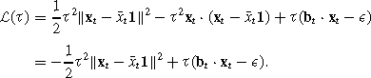Note that in the derivation of the above formula, we used the following formula, that is,

$$\Vert {\mathbf{x}}_{t} -\bar{{{x}}}_{t}{\mathbf{1}} \Vert ^{2}= {\mathbf{x}}_{t}\cdot {\mathbf{x}}_{t}-2\bar{{{x}}}_{t}({\mathbf{x}}_{t}\cdot {\mathbf{1}} )+\bar{{{x}}}_{t}^{2}({\mathbf{1}}\cdot {\mathbf{1}} )={\mathbf{x}}_{t}\cdot {\mathbf{x}}_{t}-\bar{{{x}}}_{t} ({\mathbf{x}}_{t}\cdot {\mathbf{1}} )={\mathbf{x}}_{t}\cdot ({\mathbf{x}}_{t}-\bar {{{x}}}_{t}{\mathbf{1}} ).$$

Setting the derivative of $${\mathcal{L}}(\tau)$$ with respect to τ to 0, we get,

$$0=\frac{\partial{\mathcal{L}}}{\partial\tau}= -\tau {\Vert {\mathbf{x}}_{t} -\bar{x}_{t}{\mathbf{1}} \Vert ^{2}}+{\mathbf{b}}_{t}\cdot {\mathbf{x}}_{t}-\epsilon.$$

Then τ can be set to the following formula,

$$\tau=\frac{{\mathbf{b}}_{t} \cdot {\mathbf{x}}_{t}-\epsilon}{ \Vert {\mathbf{x}}_{t} -\bar{{x}}_{t}{\mathbf{1}} \Vert ^{2}}.$$

Since τ≥0, we project τ to [0,∞), thus,

$$\tau=\max \biggl\{ 0, \frac{{\mathbf{b}}_{t} \cdot {\mathbf{x}}_{t}-\epsilon}{ \Vert {\mathbf{x}}_{t} -\bar{{x}}_{t}{\mathbf{1}} \Vert ^{2}} \biggr\}=\frac{{{\ell}_{\epsilon}^{t}}}{\Vert {\mathbf{x}}_{t} - \bar{{{x}}}_{t}{\mathbf{1}} \Vert ^{2}}.$$

Note that in case of zero market volatility, that is, $$\Vert {\mathbf{x}}_{t}-\bar{{{x}}}_{t}{\mathbf{1}} \Vert ^{2}=0$$, we just set τ=0. And we can state the update scheme for the case where $$\ell_{\epsilon}^{t}=0$$ and the case where $$\ell_{\epsilon }^{t}>0$$ by setting τ. Thus, we simplify the notation according to (1) and show the unified update scheme. □

### Proof

We derive the solution of PAMR-1 following the same procedure as the derivation of PAMR. Let us consider the situation when the loss is not 0 and we get the Lagrangian,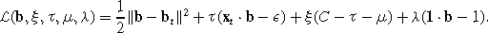Setting the partial derivatives of $${\mathcal{L}}$$ with respect to the elements of b to zero gives,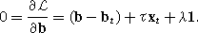Multiply both side with 1 , and b 1=1, we can get, $$\lambda= -{\tau}\frac{{\mathbf{x}}_{t} \cdot {\mathbf{1}}}{m}=-\tau{\bar{{\mathbf{x}}}_{t}}$$. And the approximation solution is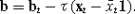Next, note that the minimum of the term ξ(Cτμ) with respect to ξ is zero whenever Cτμ=0. If Cτμ≠0 then the minimum can be made to approach −∞. Since we need to maximize the dual we can rule out the latter case and pose the following constraint on the dual variables, Cτμ=0. The KKT conditions confine μ to be non-negative so we conclude that τC. We can project τ to the interval [0,C] and get,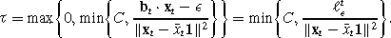Also note that we simplify the notation according to (1) and show the unified update scheme. □

### Proof

We derive the solution following the derivations of the PAMR and PAMR-1. Let us focus on the situation when the loss is not 0 and we can get the Lagrangian,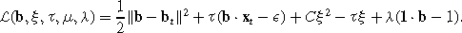Setting the partial derivatives of $${\mathcal{L}}$$ with respect to the elements of b to zero gives,Multiply both side with 1 , and b 1=1, we can get, $$\lambda= -{\tau}\frac{{\mathbf{x}}_{t} \cdot {\mathbf{1}}}{m}=-\tau{\bar{{x}}}$$. And the approximation solution is,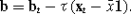Setting the partial derivatives of $${\mathcal{L}}$$ with respect to the elements of ξ to zero gives,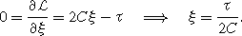Expressing ξ as above and replacing b, we rewrite the Lagrangian as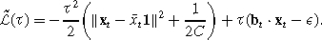Take the derivative with respect to τ and set it to zero, we can get,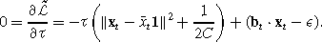Then we get the update scheme of τ, and project it to [0,∞)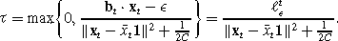□

## Rights and permissions

Reprints and Permissions

Li, B., Zhao, P., Hoi, S.C.H. et al. PAMR: Passive aggressive mean reversion strategy for portfolio selection. Mach Learn 87, 221–258 (2012). https://doi.org/10.1007/s10994-012-5281-z

• Accepted:

• Published:

• Issue Date:

• DOI: https://doi.org/10.1007/s10994-012-5281-z

### Keywords

• Portfolio selection
• Mean reversion
• Passive aggressive learning
• Online learning